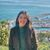# QlikView Documents

QlikView documentation and resources.

Announcements
Document boards are being consolidated, this board no longer allows NEW documents READ MORE

# Set Analysis for certain Point in Time

cancel
Showing results for
Did you mean:Luminary Alumni

## Set Analysis for certain Point in Time

Hi All,

Sometimes there may be requirements from users where they want to see the charts in from certain point of time like YTD, QTD, MTD, Last 5 Years etc., please find the set analysis expressions for this type of scenarios.

YTD Sales (Year To Date)

Sum({<Year=, Quarter=, Month=, Week=, Date={‘>=\$(=YearStart(Today()))<=\$(=Today())’}>} Sales )

Note: Year=, Quarter=, Month=, Week= excludes the selections in Year, Quarter, Month and Week dimensions.

QTD Sales (Quarter To Date)

Sum({<Year=, Quarter=, Month=, Week=, Date={‘>=\$(=QuarterStart(Today()))<=\$(=Today())’}>} Sales)

MTD Sales (Month To Date)

Sum({<Year=, Quarter=, Month=, Week=, Date={‘>=\$(=MonthStart(Today()))<=\$(=Today())’}>} Sales)

WTD Sales (Week To Date)

Sum({<Year=, Quarter=, Month=, Week=, Date={‘>=\$(=WeekStart(Today()))<=\$(=Today())’}>} Sales)

Last 5 Years Sales

Sum({<Year=, Quarter=, Month=, Week=, Date={‘>=\$(=YearStart(Today(), -4))<=\$(=Today())’}>} Sales )

Last 6 Quarters Sales

Sum({<Year=, Quarter=, Month=, Week=, Date={‘>=\$(=QuarterStart(Today(), -5))<=\$(=Today())’}>} Sales )

Last 12 Months Sales

Sum({<Year=, Quarter=, Month=, Week=, Date={‘>=\$(=MonthStart(Today(), -11))<=\$(=Today())’}>} Sales )

Last 15 Weeks Sales

Sum({<Year=, Quarter=, Month=, Week=, Date={‘>=\$(=WeekStart(Today(), -14))<=\$(=Today())’}>} Sales )

Last 10 Days Sales

Sum({<Year=, Quarter=, Month=, Week=, Date={‘>=\$(=Date(Today()-9))<=\$(=Today())’}>} Sales )

Yesterday Sales

Sum({<Year=, Quarter=, Month=, Week=, Date={‘\$(=Date(Today()-1))’}>} Sales )

You can also arrive some flags for above scenarios in script and you use those flags in Set Analysis expression if your data always based on Current Date. Refer below link created by Richard.Pearce60

Calendar with flags making set analysis so very simple

Hope this helps.

Regards,

Jagan.

Labels (5)

• ### QlikView ApplicationsNot applicable

Note: Year=, Quarter=, Month=, Week= excludes the selections in Year, Quarter, Month and Week dimensions.

What does it actual mean.....??Master III

I suggest you test it. It does exactly what it says it does: Any selections in those fields are excluded (read "ignored").Not applicable

Thanks:)

-AbdulAnonymous
Not applicable

Thank youMaster

Thanks for sharing, very usefulon daily praxisSpecialist IIPartner - Master

Thanks for the info. Good prompt.Specialist II

Well documented.Contributor III

Do you have any such Set Analysis for certain points in time for Qlik Sense Jagan? It woud be much appreciated.Partner - Contributor II

Very helpful thank you for sharing

Version history
Last update:
‎2022-09-20 01:59 PM
Updated by:Contributors